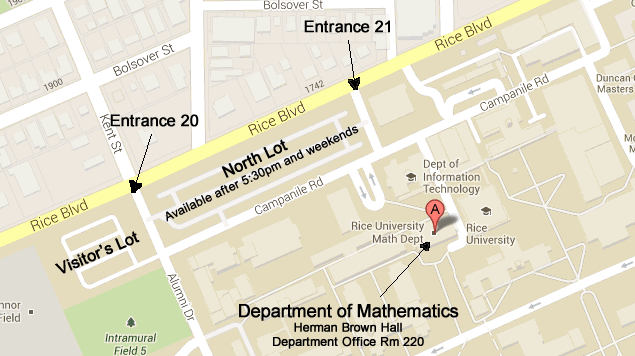# Research

The Department research interests range from differential geometry, ergodic theory, group representations, partial differential equations, and probability to real analysis, mathematical physics, complex variables, algebraic geometry, number theory, combinatorics, geometric topology, algebraic topology, and dynamics.Large Map

Tel (713) 348-4829